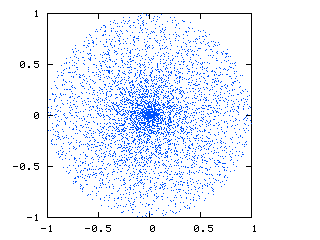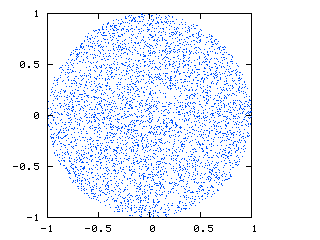When adapting combinatorial metaheuristics to continuous problems, one sometimes use a sphere as an approximation of the "neighborhood". The idea is thus to draw the neighbours around a solution, for example in order to apply a simple mutation in a genetic algorithm.

Sometimes, one choose to use an uniform law, but how to draw random vectors uniformly in an hyper-sphere ?

The first idea that comes to mind is to use a polar coordinate system and draw the radius r and the angles a1...a2...ai...aN with a uniform law. Then, one convert the coordinates in the rectangular system, x1...x2...xi...xN.

The result is interesting for a metaheuristic design, but is not a uniform distribution:The correct method is to draw each xi according to: xi=(ri1/Nai)/√(∑(ai))
(in LATEX : $x_{i}=\frac{r^{\frac{1}{N}}_i\cdot a_{i}}{\sqrt{\sum _{j=1}^{N}a_{i}}}$)

With ri uniformly drawn in U0,1 and a following a normal law NO,1

The result is then a true uniform distribution:Credits goes to Maurice Clerc for detecting and solving the problem.